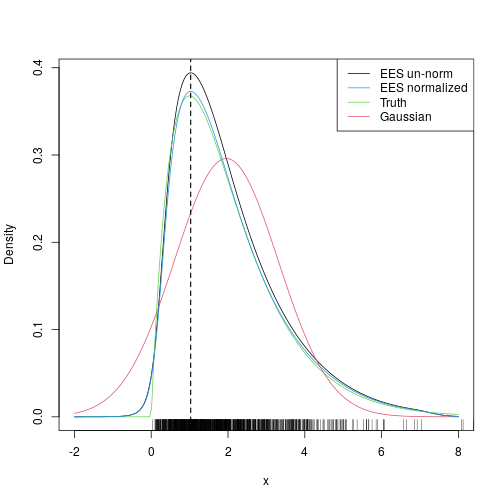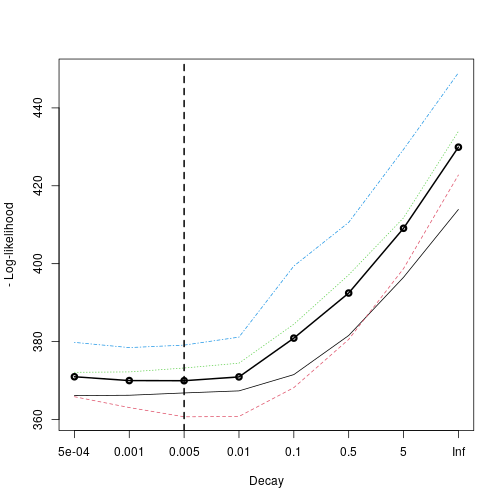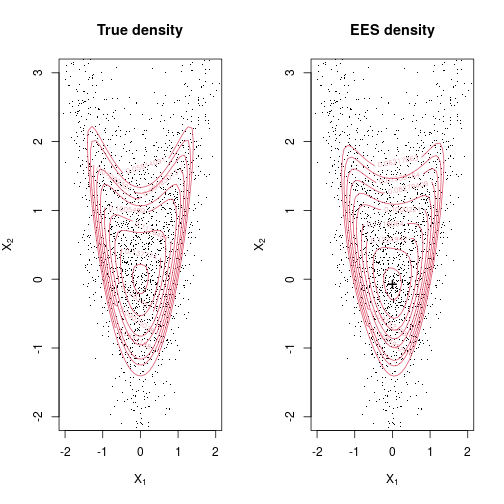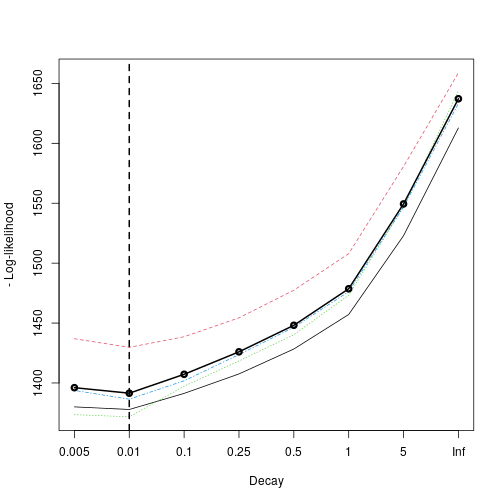## Introduction

This package implements the Extended Empirical Saddlepoint density approximation (EES) proposed by Fasiolo et al., 2016.

The main functions are:

• dsaddle(): evaluates the EES density, given some data.
• selectDecay(): selects the tuning parameter of EES by cross-validation.
• findMode(): maximizes EES in order to find its mode.

Here we describe how to use them with two simple examples.

## An univariate example

Here we use the EES density to approximate an univariate Gamma(2, 1) density. We simulate some Gamma(2, 1) random variables, and then we compare the true density, a Gaussian fit, the un-normalized EES density and a normalized EES density. The normalizing constant is computed using 500 importance samples.

library(esaddle)
set.seed(4141)
x <- rgamma(1000, 2, 1)

# Fixing tuning parameter of EES
decay <-  0.05

# Evaluating EES at several point
xSeq <- seq(-2, 8, length.out = 200)
tmp <- dsaddle(y = xSeq, X = x, decay = decay, log = TRUE)  # Un-normalized EES
tmp2 <- dsaddle(y = xSeq, X = x, decay = decay,             # EES normalized by importance sampling
normalize = TRUE, control = list("method" = "IS", nNorm = 500), log = TRUE)

# Plotting true density, EES and normal approximation
plot(xSeq, exp(tmp$llk), type = 'l', ylab = "Density", xlab = "x") lines(xSeq, dgamma(xSeq, 2, 1), col = 3) lines(xSeq, dnorm(xSeq, mean(x), sd(x)), col = 2) lines(xSeq, exp(tmp2$llk), col = 4)
suppressWarnings( rug(x) )
legend("topright", c("EES un-norm", "EES normalized", "Truth", "Gaussian"), col = c(1, 4, 3, 2), lty = 1)
res <- findMode(x, init = mean(x), decay = decay)$mode abline(v = res, lty = 2, lwd = 1.5)Notice that, upon normalization, the ESS gives an almost perfect fit. Also, in the last two lines we have used findMode() to find EES's mode, whose location obviously does not depend on the normalizing constant. Above we have determined the EES's tuning parameter, decay, manually but this can be chosen by k-fold cross-valdation using selectDecay(), that is: tmp <- selectDecay(decay = c(5e-4, 1e-3, 5e-3, 0.01, 0.1, 0.5, 5, Inf), # grid of decay values K = 4, simulator = function() x, multicore = T, ncores = 2)Here we were using four folds, and we distributed the computation on two cores. The score on the vertical axis is the negative log-likelihood on the out-of-fold data. The speed at which EES converges to a Gaussian density fit, as we moves toward the tails, is directly proportional to decay. Here it looks like we will decay toward the normal density fairly slowly (the optimal decay is low), which makes sense because the distribution the random variables in x is far from normal. ## A bivariate example Here we consider a banana-shaped or warped bivariate Gaussian density. We firstly define its density and a function that simulates random variables: dwarp <- function(x, alpha) { lik <- dnorm(x[ , 1], log = TRUE) tmp <- x[ , 1]^2 lik <- lik + dnorm(x[ , 2] - alpha*tmp, log = TRUE) lik } rwarp <- function(n = 1, alpha) { z <- matrix(rnorm(n*2), n, 2) tmp <- z[ , 1]^2 z[ , 2] <- z[ , 2] + alpha*tmp z }  The distribution is quite simple: $$\alpha$$ is a constant, $$x_1 \sim N(0, 1)$$ and $$x_2 = z + \alpha x_1$$, where $$z \sim N(0, 1)$$. We simulate some random variables and then we evaluate the true density and the EES approximation on a grid of points: alpha <- 1 X <- rwarp(2000, alpha = alpha) # Creating 2d grid m <- 50 expansion <- 1 x1 <- seq(-2, 3, length=m)* expansion; x2 <- seq(-3, 3, length=m) * expansion x <- expand.grid(x1, x2) # Evaluating true density on grid alpha <- 1 dw <- exp( dwarp(x, alpha = alpha) ) # Evaluating EES density dwa <- dsaddle(as.matrix(x), X, decay = 0.05, log = FALSE)$llk


We then plot true and EES density and add the mode of EES (black cross) to the plot:

# Plotting true density
par(mfrow = c(1, 2))
plot(X, pch=".", col=1, ylim = c(-2, 3), xlim = c(-2, 2),
main = "True density", xlab = expression(X), ylab = expression(X))
contour(x1, x2, matrix(dw, m, m), levels = quantile(as.vector(dw), seq(0.8, 0.995, length.out = 10)), col=2, add=T)

# Plotting EES density
plot(X, pch=".",col=1, ylim = c(-2, 3), xlim = c(-2, 2),
main = "EES density", xlab = expression(X), ylab = expression(X))
contour(x1, x2, matrix(dwa, m, m), levels = quantile(as.vector(dwa), seq(0.8, 0.995, length.out = 10)), col=2, add=T)

# Finding mode using EES
init <- rnorm(2, 0, sd = c(1, 2)) # random initialization
res <- findMode(X = X, init = init, decay = 0.05)\$mode
points(res, res, pch = 3, lwd = 2)As in the previous example, the decay tuning parameter could be determined by cross-validation, rather than manually. Here we use four folds and two cores:

tmp <- selectDecay(decay = c(0.005, 0.01, 0.1, 0.25, 0.5, 1, 5, Inf),
K = 4,
simulator = function() X,
multicore = T,
ncores = 2)## References

• Fasiolo, M., Wood, S. N., Hartig, F. and Bravington, M. V. (2016). An Extended Empirical Saddlepoint Approximation for Intractable Likelihoods. URL: ArXiv https://arxiv.org/abs/1601.01849.
h